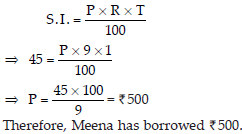# Comparing Quantities (Maths) Class 7 - NCERT Questions

Q 1.

Find the ratio of :
(A) Rs. 5 to 50 paise
(B) 15 kg to 210 g
(C) 9 m to 27 cm
(D) 30 days to 36 hours

SOLUTION: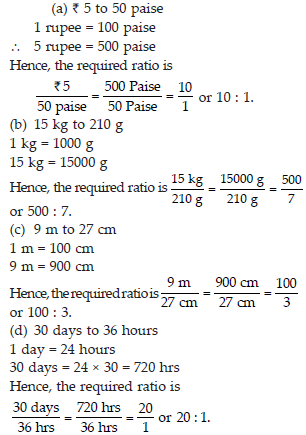Q 2.

In a computer lab, there are 3 computers for every 6 students. How many computers will be needed for 24 students?

SOLUTION: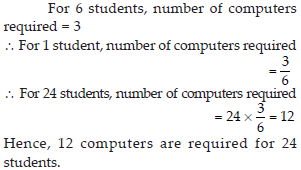Q 3.

Population of Rajasthan = 570 lakhs and population of UP = 1660 lakhs.
Area of Rajasthan = 3 lakhs km2 and area of UP = 2 lakhs km2
(i) How many people are there per km2 in both these states?
(ii) Which state is less populated?

SOLUTION: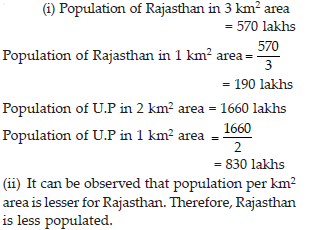Q 4.

Convert the given fractional numbers to percents.
(A) 1/8
(B) 5/4
(C) 3/40
(D) 2/7

SOLUTION: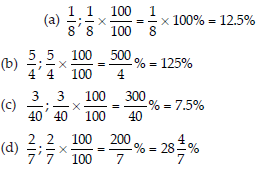Q 5.

Convert the given decimal fractions to percents. (A) 0.65
(B) 2.1
(C) 0.02
(D) 12.35

SOLUTION: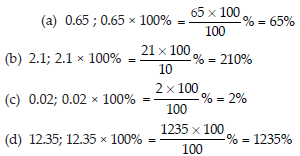Q 6.

Estimate what part of the figures is coloured and hence find the per cent which is coloured.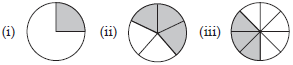SOLUTION: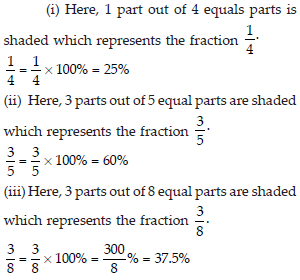Q 7.

Find :
(A) 15% of 250
(B) 1% of 1 hour
(C) 20% of Rs. 2500
(D) 75% of 1 kg

SOLUTION: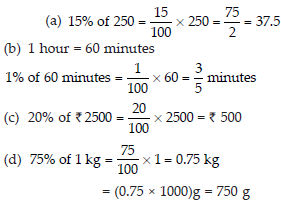Q 8.

Find the whole quantity if
(A) 5% of it is 600
(B) 12% of it is Rs. 1080
(C) 40% of it is 500 km
(D) 70% of it is 14 minutes
(E) 8% of it is 40 litres

SOLUTION: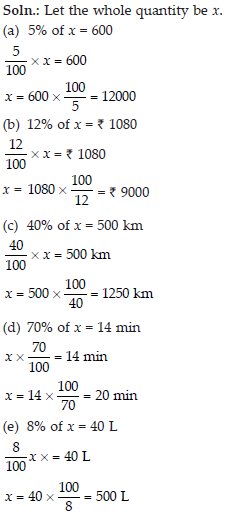Q 9.

Convert given percents to decimal fractions and also to fractions in simplest forms:
(A) 25%
(B) 150%
(C) 20%
(D) 5%

SOLUTION: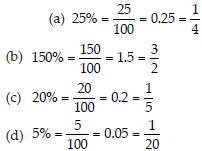Q 10.

In a city, 30% are females, 40% are males and remaining are children. What per cent are children?

SOLUTION: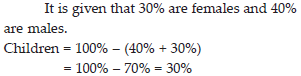Q 11.

Out of 15,000 voters in a constituency, 60% voted. Find the percentage of voters who did not vote. Can you now find how many actually did not vote?

SOLUTION: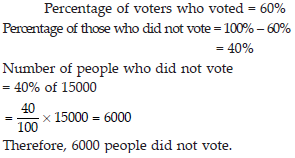Q 12.

Meeta saves Rs. 400 from her salary. If this is 10% of her salary. What is her salary?

SOLUTION: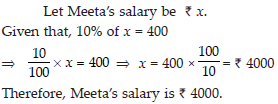Q 13.

A local cricket team played 20 matches in one season. It won 25% of them. How many matches did they win?

SOLUTION: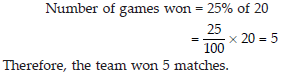Q 14.

Tell what is the profit or loss in the following transactions. Also find profit percent or loss percent in each case.
(A) Gardening shears bought for Rs. 250 and sold for Rs. 325.
(B) A refrigerator bought for Rs. 12,000 and sold at Rs. 13,500.
(C) A cupboard bought for Rs. 2,500 and sold at Rs. 3,000.
(D) A skirt bought for Rs. 250 and sold at Rs. 150.

SOLUTION: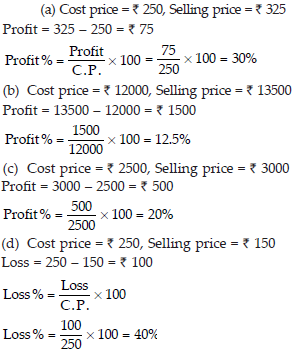Q 15.

Convert each part of the ratio to percentage:
(A) 3 : 1
(B) 2 : 3 : 5
(C) 1 : 4
(D) 1 : 2 : 5

SOLUTION: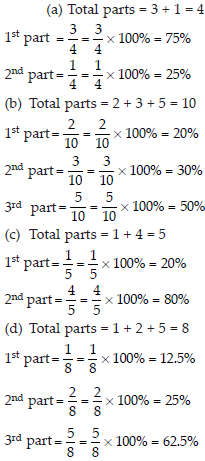Q 16.

The population of a city decreased from 25,000 to 24,500. Find the percentage decrease.

SOLUTION: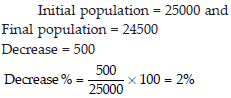Q 17.

Arun bought a car for Rs. 3,50,000. The next year, the price went upto Rs. 3,70,000. What was the percentage of price increase?

SOLUTION: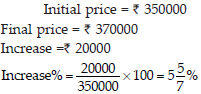Q 18.

I buy a T.V. for Rs.10,000 and sell it at a profit of 20%. How much money do I get for it?

SOLUTION: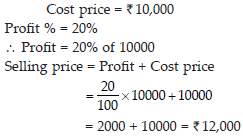Q 19.

Juhi sells a washing machine for Rs.13,500. She loses 20% in the bargain. What was the price at which she bought it?

SOLUTION: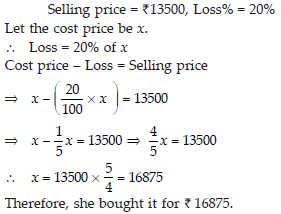Q 20.

(i) Chalk contains calcium, carbon and oxygen in the ratio 10 : 3 : 12. Find the percentage of carbon in chalk.
(ii) If in a stick of chalk, carbon is 3g, what is the weight of the chalk stick?

SOLUTION: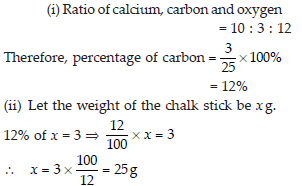Q 21.

Amina buys a book for Rs.275 and sells it at a loss of 15%. How much does she sell it for?

SOLUTION: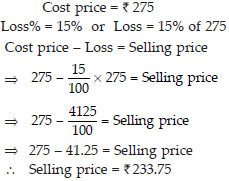Q 22.

Find the amount to be paid at the end of 3 years in each case:
(A) Principal = Rs.1,200 at 12% p.a.
(B) Principal = Rs. 7,500 at 5% p.a.

SOLUTION: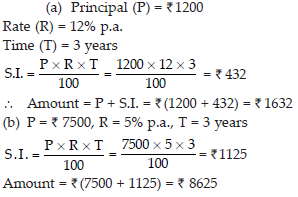Q 23.

What rate gives Rs. 280 as interest on a sum of Rs. 56,000 in 2 years?

SOLUTION: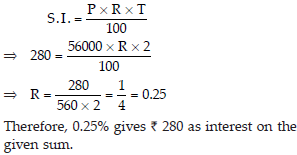Q 24.

If Meena gives an interest of Rs.45 for one year at 9% rate p.a.. What is the sum she has borrowed?

SOLUTION: